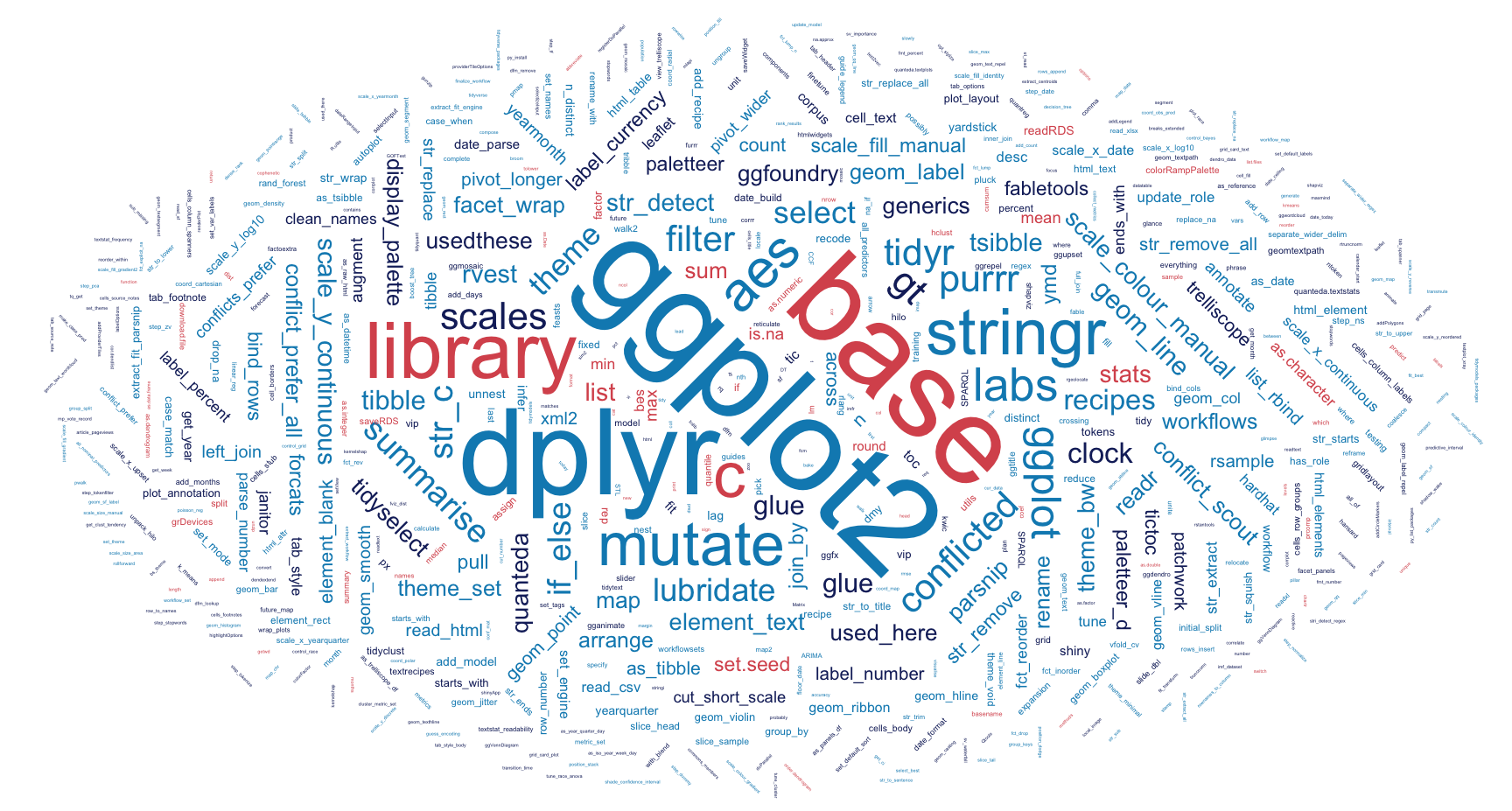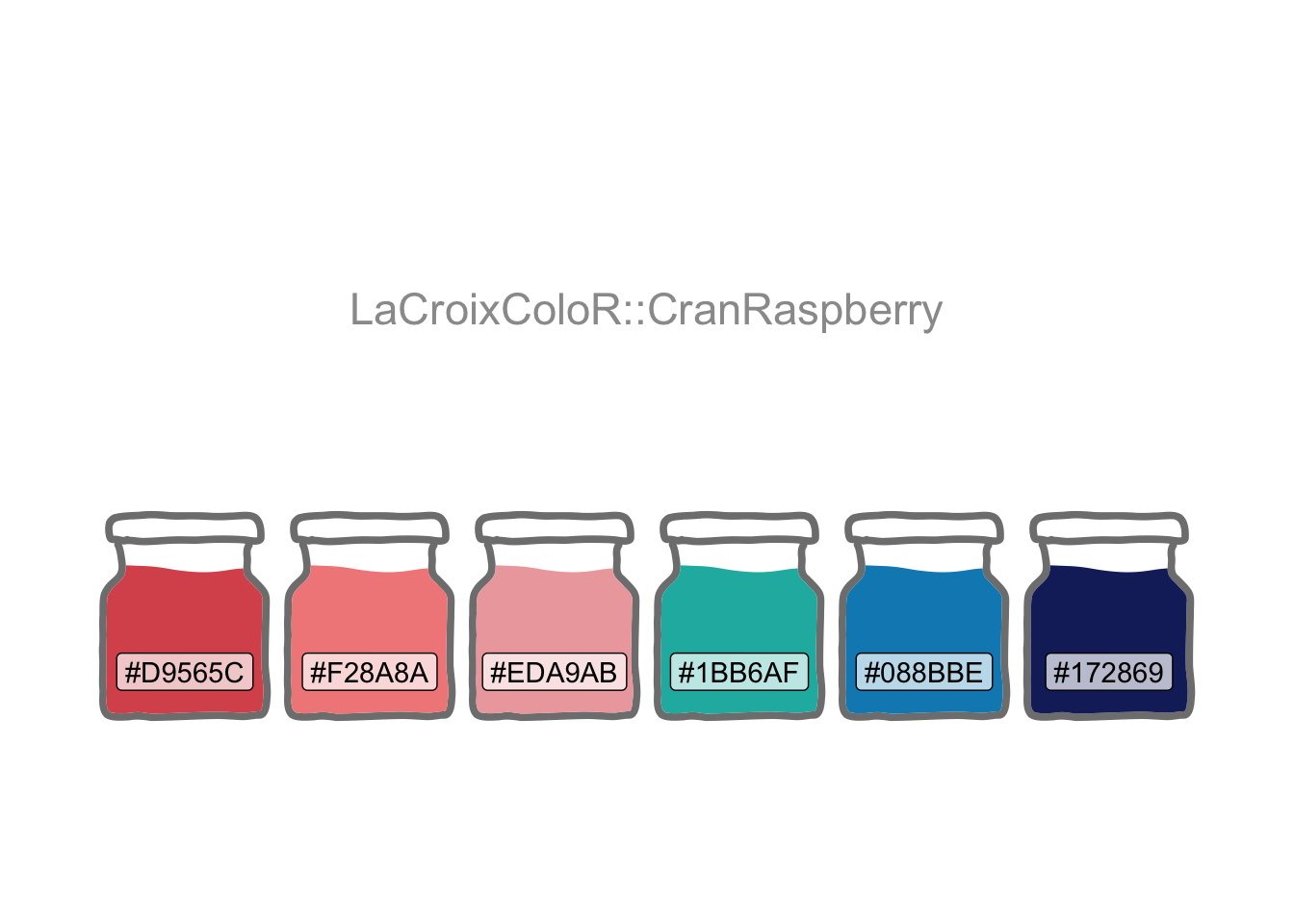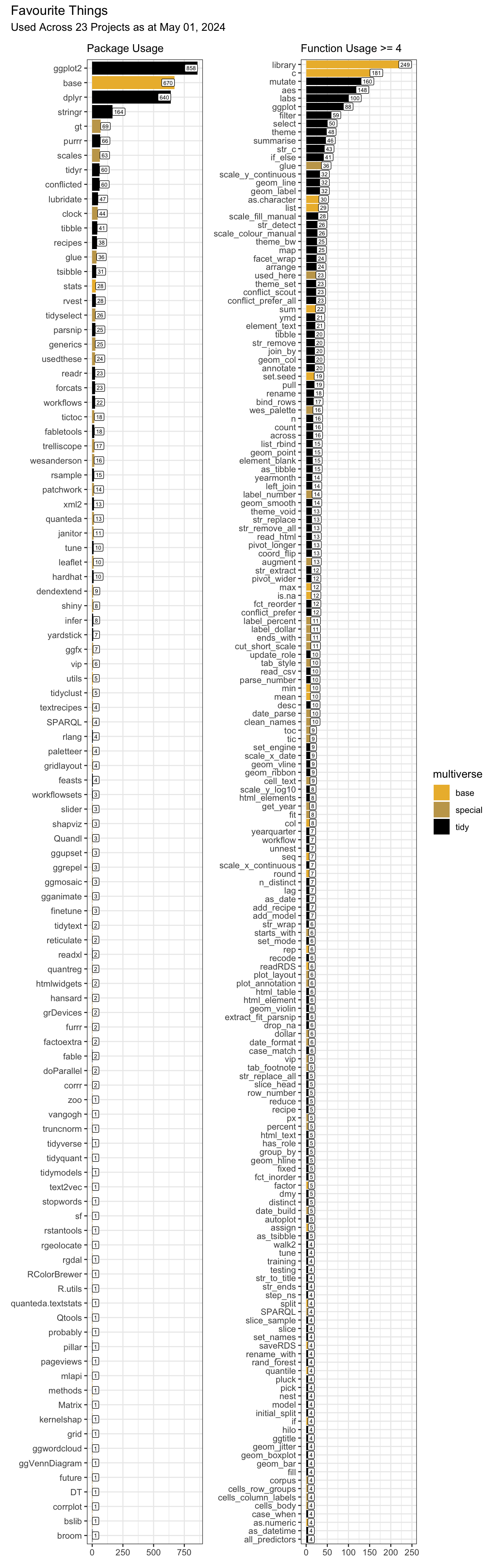# Favourite Things

R
web scraping
R packages & functions that make doing data science a joy based on usage across projects
Author

Carl Goodwin

Published

July 26, 2020

Modified

June 12, 2023Each project closes with a table summarising the R tools used. By aggregating the package and function usage across all projects, there’s an opportunity to:

• Spot the use of superseded functions like `map_dfr`

• Check for usage consistency, e.g. `clock::date_today` versus `Sys.Date`

• See where it would be most useful to keep an eye on package version updates

Since starting this in 2017, functions like tidyr’s `spread` and `gather` have been superseded by `pivot_wider` and `pivot_longer`. Newer packages have emerged like tidyclust, which brings cluster modelling to tidymodels (now used in Finding Happiness in ‘The Smoke’). bslib has brought improvements to the latest shiny app version embedded in Plots Thicken. The paletteer package has put it’s arms around the myriad palette packages out there. And scales’ `cut_short_scale` assisted with plot labelling.

Most recently, the latest versions of dplyr and purrr have presented a host of enhancements. For example, the `.by` argument in `mutate` and friends offers a neat alternative to `group_by` and `ungroup` in many situations. Joins have been enhanced (e.g. to support inequality conditions) with the addition of `join_by` to dplyr. And changes in the `map_` family introduce `list_rbind` and associates with `map_dfr`, for example, now superseded.

``````library(conflicted)
library(tidyverse)
conflict_prefer_all("dplyr", quiet = TRUE)
library(tidytext)
library(rvest)
library(paletteer)
library(janitor)
library(glue)
library(ggwordcloud)
library(patchwork)
library(clock)
library(usedthese)

conflict_scout()``````
``````theme_set(theme_bw())

n <- 4
palette <- "harrypotter::hufflepuff"

cols <- paletteer_c(palette, n = n)

tibble(x = 1:n, y = 1) |>
ggplot(aes(x, y, fill = cols)) +
geom_col(colour = "white") +
geom_label(aes(label = cols |> str_remove("FF\$")),
size = 4, vjust = 2, fill = "white") +
annotate(
"label",
x = (n + 1) / 2, y = 0.5,
label = palette,
fill = "white",
alpha = 0.8,
size = 6
) +
scale_fill_manual(values = as.character(cols)) +
theme_void() +
theme(legend.position = "none")``````Separation of tidyverse and non-tidyverse packages may be achieved by using the likes of `tidyverse_packages` which lists all packages in the tidyverse.

``````tidy <-
c(
tidyverse::tidyverse_packages(),
fpp3::fpp3_packages(),
tidymodels::tidymodels_packages()
) |>
unique()``````

`used_here()` has already been used in Quantum Jitter projects to generate a usage table at the foot of each project with the CSS class usedthese. `used_there()` may now be used to web-scrape all the tables with this class to aggregate package and function usage data.

``````base_packages <- c(
"stats",
"graphics",
"grDevices",
"utils",
"datasets",
"methods",
"base"
)

used_df <-
used_there("https://www.quantumjitter.com/project/") |>
mutate(multiverse = case_match(
Package,
tidy ~ "tidy",
base_packages ~ "base",
.default = "special"
))

n_url <- used_df |> summarise(n_distinct(url)) |> pull()

pack_df <- used_df |>
count(Package, multiverse, wt = n) |>
mutate(name = "package")

fun_df <- used_df |>
count(Function, multiverse, wt = n) |>
mutate(name = "function")

packfun_df <- pack_df |>
bind_rows(fun_df) |>
arrange(desc(n)) |>
mutate(
packfun = coalesce(Package, Function),
name = fct_rev(name),
.by = name
)``````

With the latest versions of dplyr and purrr, usage of `group_by`, `ungroup` and `map_dfr` has fallen away whilst usage of `list_rbind` and `join_by` has freshly emerged.

``````p1 <- packfun_df |>
filter(name == "package") |>
ggplot(aes(fct_reorder(packfun, n), n, fill = multiverse)) +
geom_col(show.legend = FALSE) +
coord_flip() +
geom_label(aes(label = n), hjust = "inward", size = 2, fill = "white") +
scale_fill_manual(values = cols[c(4, 3, 1)]) +
labs(x = NULL, y = NULL,
subtitle = glue("Package Usage"))

min_n <- 4

p2 <- packfun_df |>
filter(name == "function", n >= min_n) |>
ggplot(aes(fct_reorder(packfun, n), n, fill = multiverse)) +
geom_col() +
coord_flip() +
geom_label(aes(label = n), hjust = "inward", size = 2, fill = "white") +
scale_fill_manual(values = cols[c(4, 3, 1)]) +
labs(x = NULL, y = NULL,
subtitle = glue("Function Usage >= {min_n}"))

p1 + p2 +
plot_annotation(
title = glue("Favourite Things"),
subtitle = glue(
"Used Across {n_url} Projects as at ",
"{date_format(date_today('Europe/London'), format = '%B %d, %Y')}"
)
)``````This last code chunk generates the word cloud for use as the feature image for this project.

``````set.seed = 123

packfun_df |>
mutate(angle = 45 * sample(-2:2, n(),
replace = TRUE,
prob = c(1, 1, 4, 1, 1))) |>
ggplot(aes(
label = packfun,
size = n,
colour = multiverse,
angle = angle
)) +
geom_text_wordcloud(
eccentricity = 1,
grid_margin = 0.95,
seed = 789
) +
scale_size_area(max_size = 20) +
scale_colour_manual(values = cols[c(4, 2, 1)]) +
theme_void() +
theme(plot.background = element_rect(fill = cols))``````## R Toolbox

This project’s code too should be included in my “favourite things”.

``used_here()``
Package Function
base as.character, c, library, sample, unique
clock date_format, date_today
conflicted conflict_prefer_all, conflict_scout
dplyr arrange, bind_rows, case_match, coalesce, count, desc, filter, mutate, n, n_distinct, pull, summarise
forcats fct_reorder, fct_rev
ggplot2 aes, annotate, coord_flip, element_rect, geom_col, geom_label, ggplot, labs, scale_colour_manual, scale_fill_manual, scale_size_area, theme, theme_bw, theme_set, theme_void
ggwordcloud geom_text_wordcloud
glue glue
paletteer paletteer_c
patchwork plot_annotation
stringr str_remove
tibble tibble
tidymodels tidymodels_packages
tidyverse tidyverse_packages
usedthese used_here, used_there